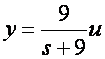## A Simple Kalman Filter in Simulink

This tutorial presents a simple example of how to implement a Kalman filter in Simulink. If you are unfamiliar with the mathematics behind the Kalman filter then see this tutorial.

A Simulink model that implements a simple Kalman Filter using an Embedded MATLAB Function block is shown in Figure 1. The purpose of this model is to show how a Kalman Filter may be used to identify any unmeasured states of a discrete time process using only the process input signal and a noisy measured output..Figure 1: Simulink Model for implementing a Kalman Filter.

The discrete process under consideration is a simple first order, time-invariant system given by the following set of equations,Note that the above model represents a discrete version of the continuous time transfer function,where the discrete sample rate is Ts = 0.01. Hence the model should be expected to track a step input with zero steady-state error.

The process model is subject to two sources of zero-mean Gaussian noise. The input is corrupted with noise having a variance of 0.01 and the output is corrupted with noise having a variance of 0.1.

As discussed in a previous tutorial the Kalman filter uses a predictor-corrector algorithm to estimate the internal state of the discrete process. Note that for this model the state and the output are scaled versions of each other so the terms can be used interchangeably. Hence although both the estimated state and output have been calculated by the Kalman Filter only the estimated output is being displayed on the Scope.

The input signal, measured output and estimated output are displayed on the Scope. This is shown in Figure 2.

Note that the Kalman filter does a good job of rejecting the noise and estimating a signal that accurately tracks the step input.Figure 2: Step Response.

The Kalman Filter itself has been implemented in an Embedded MATLAB Function block. The code within that block is shown below.

```function [xhatOut, yhatOut] = KALMAN(u,meas)
% This Embedded MATLAB Function implements a very simple Kalman filter.
%
% It implements a Kalman filter for estimating both the state and output
% of a linear, discrete-time, time-invariant, system given by the following
% state-space equations:
%
% x(k) = 0.914 x(k-1) + 0.25 u(k) + w(k)
% y(k) = 0.344 x(k-1) + v(k)
%
% where w(k) has a variance of 0.01 and v(k) has a variance of 0.1.

% Author: Phil Goddard (phil@goddardconsulting.ca)
% Date: Q2, 2011.

% Define storage for the variables that need to persist
% between time periods.
persistent P xhat A B C Q R
if isempty(P)
% First time through the code so do some initialization
xhat = 0;
P = 0;
A = 0.914;
B = 0.25;
C = 0.344;
Q = 0.01^2;
R = 0.1^2;
end
% Propagate the state estimate and covariance matrix:
xhat = A*xhat + B*u;
P = A*P*A' + Q;
% Calculate the Kalman gain
K = P*C'/(C*P*C' + R);
% Calculate the measurement residual
resid = meas - C*xhat;
% Update the state and error covariance estimate
xhat = xhat + K*resid;
P = (eye(size(K,1))-K*C)*P;
% Post the results
xhatOut = xhat;
yhatOut = C*xhatOut;
```

The main purpose of this tutorial is to demonstrate a Simulink implementation of the Kalman Filter equations. The process model under consideration has deliberately been chosen to be very simple, and consequently the Kalman filter does a good job at rejecting the process and measurement noise to generate a very good estimate of the process output. Other tutorials discuss the application of the Kalman Filter to estimating the states of more complex systems, and its use in estimating unknown model parameters.